Home > INT3 > Chapter 11 > Lesson 11.1.4 > Problem11-46

11-46.
1. Without using a calculator, write two solutions to each of the following equations for 0º ≤ θ < 360º. Homework Help ✎

1. sin(θ) =2. tan(θ) =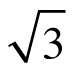3. cos(θ) =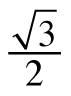4. sin(θ) = −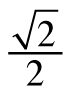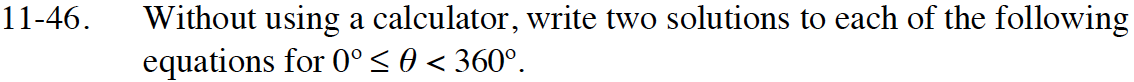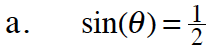One answer is θ = 30°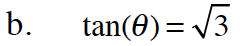Tangent is positive in quadrants I and III.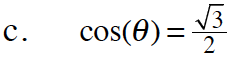θ = 30°, 330°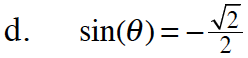Sine is negative in quadrants III and IV.